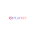## Tuesday, April 30, 2013

### Distribution of The Difference of Two Uniform Distribution Variable

Suppose U1~uniform(0,1), U2~uniform(0,1), and U1 is independent of U2, then what is the distribution of U1-U2?

The solution is in the picture attached. Then based on this, what is the distribution of U1+U2? The density should be of the same shape while it moves 1 unit to the right. The reason is: if U2 is uniform, then U3=1-U2 is uniform. so U1+U2 is the same as U1+U2=U1-U3+1. So it's density is the same as U1-U2 with i unit right transfer.

The answer can be verified in R. Density plot in R:
```u1=runif(1000000,0,1)
u2=runif(1000000,0,1)

# Z is the difference of the two uniform distributed variable
z=u1-u2
plot(density(z), main="Density Plot of the Difference of Two Uniform Variable", col=3)

# X is the summation of the two uniform distributed variable
x=u1+u2
plot(density(x), main="Density Plot of the Summation of Two Uniform Variable", col=3)```

1.Thank you for the nice article here. Really nice and keep update to explore more gaming tips and ideas.

Game Testing Company

Video Game Testing Company

Mobile Game Testing

Focus Group Testing

Game QA Testing

2.3.Very useful post and I think it is rather easy to see from the other comments as well that this post is well written and useful.Keep up the good work
software testing companies
QA testing services
software testing and quality assurance services

4.playrep is offer you to play lots of games like - fungame, funrep, playrep etc..

5.6.7.8.